This case study uses Stan to fit the Rasch and two-parameter logistic (2PL) item response theory models, including a latent regression for person ability for both. The Rasch model is some times referred to as the one-parameter logistic model. Analysis is performed with R, making use of the rstan and edstan packages. rstan is the implementation of Stan for R, and edstan provides Stan models for item response theory and several convenience functions.

The edstan package is available on CRAN, but a more up to date version may often be found on Github. The following R code may be used to install the package from Github.

# Install edstan from Github rather than CRAN
install.packages("devtools")
devtools::install_github("danielcfurr/edstan")

The following R code loads the necessary packages and then sets some rstan options, which causes the compiled Stan model to be saved for future use and the MCMC chains to be executed in parallel.

# Load R packages
library(rstan)
library(edstan)
library(ggplot2)
library(TAM)
rstan_options(auto_write = TRUE)
options(mc.cores = parallel::detectCores())

The case study uses R version 3.3.2, rstan version 2.14.1, ggplot2 version 2.2.1, and edstan version 1.0.7. Also, the example data are from TAM version 1.995.0. Readers may wish to check the versions for their installed packages using the packageVersion() function.

# 1 Rasch model with latent regression

## 1.1 Overview of the model

The Rasch model (Rasch 1960) is an item response theory model for dichotomous items. The version presented includes a latent regression, in which the person abilities are regressed on person characteristics. However, the latent regression part of the model may be restricted to an intercept only, resulting in a regular Rasch model.

$\mathrm{logit} [ \Pr(y_{ij} = 1 | \theta_j, \beta_i) ] = \theta_j - \beta_i$ $\theta_j \sim \mathrm{N}(w_j' \lambda, \sigma^2)$

Variables:

• $$i = 1 \ldots I$$ indexes items.
• $$j = 1 \ldots J$$ indexes persons.
• $$y_{ij} \in \{ 0, 1 \}$$ is the response of person $$j$$ to item $$i$$.
• $$w_{j}$$ is the vector of covariates for person $$j$$, the first element of which must equal one for a model intercept. $$w_{j}$$ may be assembled into a $$J$$-by-$$K$$ covariate matrix $$W$$, where $$K$$ is number of elements in $$w_j$$.

Parameters:

• $$\beta_i$$ is the difficulty for item $$i$$.
• $$\theta_j$$ is the ability for person $$j$$.
• $$\lambda$$ is the vector of latent regression parameters of length $$K$$.
• $$\sigma^2$$ is the variance for the ability distribution.

Constraints:

• The last item difficulty is constrained to be the negative sum of the other difficulties, $$\beta_I = -\sum_{i}^{(I-1)} \beta_i$$, resulting in the average item difficulty being zero.

Priors:

• $$\sigma \sim \mathrm{Exp}(.1)$$ is weakly informative for the person standard deviation.
• $$\beta_i \sim \mathrm{N}(0, 9)$$ is also weakly informative.
• $$\lambda \sim t_3(0, 1)$$, where $$t_3$$ is the Student’s $$t$$ distribution with three degrees of freedom, and the covariates have been transformed as follows: (1) continuous covariates are mean-centered and then divided by two times their standard deviations, (2) binary covariates are mean-centered and divided their maximum minus minimum values, and (3) no change is made to the constant, set to one, for the model intercept. This approach to setting priors is similar to one that has been suggested for logistic regression (Gelman et al. 2008). It is possible to adjust the coefficients back to the scales of the original covariates.

## 1.2Stan code for a simple Rasch model

A simple Stan model is described before discussing the complete model, as the code for the complete model is somewhat cumbersome. The simpler model, printed below, omits the latent regression and so does not require rescaling of the person covariates or lambda. The mean of the person distribution is set to zero and the constraint is removed from the item difficulties, which also differs from the complete model.

# Print the simple Rasch model from the edstan package
simple_rasch_file <- system.file("extdata/rasch_simple.stan",
package = "edstan")
cat(readLines(simple_rasch_file), sep = "\n")
data {
int<lower=1> I;               // # questions
int<lower=1> J;               // # persons
int<lower=1> N;               // # observations
int<lower=1, upper=I> ii[N];  // question for n
int<lower=1, upper=J> jj[N];  // person for n
int<lower=0, upper=1> y[N];   // correctness for n
}
parameters {
vector[I] beta;
vector[J] theta;
real<lower=1> sigma;
}
model {
beta ~ normal(0, 3);
theta ~ normal(0, sigma);
sigma ~ exponential(.1);
y ~ bernoulli_logit(theta[jj] - beta[ii]);
}

Data are fed into the model in vector form. That is, y is a long vector of scored item responses, and ii and jj indicate with which item and person each element in y is associated. These three vectors are of length N, which is equal to I times J if there are no missing responses. Parameters beta, theta, and sigma are declared in the parameters block, and priors for these are set in the model block. The likelihood for y in the last line uses vectorization by indexing theta and beta with jj and ii, which is more efficient than using a loop.

## 1.3Stan code for the Rasch model with latent regression

The Rasch model with latent regression, which is featured in edstan, is printed below. It is more complicated than is typically necessary for a Stan model because it is written to apply sensible priors for regression parameters associated with arbitrarily scaled covariates.

# Print the latent regression Rasch model from the edstan package
rasch_latreg_file <- system.file("extdata/rasch_latent_reg.stan",
package = "edstan")
cat(readLines(rasch_latreg_file), sep = "\n")
functions {
matrix obtain_adjustments(matrix W) {
real min_w;
real max_w;
int minmax_count;
matrix[2, cols(W)] adj;
adj[1, 1] = 0;
adj[2, 1] = 1;
if(cols(W) > 1) {
for(k in 2:cols(W)) {                       // remaining columns
min_w = min(W[1:rows(W), k]);
max_w = max(W[1:rows(W), k]);
minmax_count = 0;
for(j in 1:rows(W))
minmax_count = minmax_count + W[j,k] == min_w || W[j,k] == max_w;
if(minmax_count == rows(W)) {       // if column takes only 2 values
adj[1, k] = mean(W[1:rows(W), k]);
adj[2, k] = (max_w - min_w);
} else {                            // if column takes > 2 values
adj[1, k] = mean(W[1:rows(W), k]);
adj[2, k] = sd(W[1:rows(W), k]) * 2;
}
}
}
return adj;
}
}
data {
int<lower=1> I;               // # questions
int<lower=1> J;               // # persons
int<lower=1> N;               // # observations
int<lower=1, upper=I> ii[N];  // question for n
int<lower=1, upper=J> jj[N];  // person for n
int<lower=0, upper=1> y[N];   // correctness for n
int<lower=1> K;               // # person covariates
matrix[J,K] W;                // person covariate matrix
}
transformed data {
matrix[2,K] adj;               // values for centering and scaling covariates
matrix[J,K] W_adj;             // centered and scaled covariates
adj = obtain_adjustments(W);
for(k in 1:K) for(j in 1:J)
W_adj[j,k] = (W[j,k] - adj[1,k]) / adj[2,k];
}
parameters {
vector[I-1] beta_free;
vector[J] theta;
real<lower=0> sigma;
vector[K] lambda_adj;
}
transformed parameters {
vector[I] beta;
beta[1:(I-1)] = beta_free;
beta[I] = -1*sum(beta_free);
}
model {
target += normal_lpdf(beta | 0, 3);
theta ~ normal(W_adj*lambda_adj, sigma);
lambda_adj ~ student_t(3, 0, 1);
sigma ~ exponential(.1);
y ~ bernoulli_logit(theta[jj] - beta[ii]);
}
generated quantities {
vector[K] lambda;
lambda[2:K] = lambda_adj[2:K] ./ to_vector(adj[2,2:K]);
lambda = W_adj[1, 1:K]*lambda_adj[1:K] - W[1, 2:K]*lambda[2:K];
}

The Stan model begins with the creation of the obtain_adjustments() function, which accepts a covariate matrix and returns a matrix that contains values to be used in adjusting the covariates. The returned matrix has one column for each covariate (starting with the constant for the intercept). The first row of it provides the values used to center the covariates, and the second provides the values used to scale them. The function begins by setting the values in the first column to zero and one, which corresponds to no change to the constant. Next the function loops over the remaining columns of the covariate matrix and determines whether the covariate is binary or continuous. This determination is made by counting the number of values that are equal to either the maximum or minimum for a given coavariate; if this count equals $$J$$, then the covariate must be binary. Based on this determination the appropriate adjustments for the covariates are calculated and then added to the returned matrix.

In the transformed data block, the obtain_adjustments() function is called and the results are stored in adj. Then a double loop is used to assign adjusted covariate values to W_adj using W and adj. The latent regression is carried out in the model block in the declaration of the prior for theta, based on W_adj and lambda_adj. (This approach is referred to as hierarchical centering and tends to be more efficient when there is a large amount of data. The alternative is a “decentered” approach in which the prior mean for theta would be set to zero, and then W*lambda would be added to theta in the likelihood statement.)

The generated quantities block is used to calculate what the regression coefficients and intercept would have been on the scales of the original covariates. For the coefficients this is determined simply by dividing the coefficients by the same value of spread used to modify the scale the original covariates. The intercept given the original scale is then recovered with some algebra. The obtain_adjustments() function and related code for adjusting the covariates and regression coefficients is used in the same way across edstan models.

There are a few other differences from the simple model. In the data block, the number of covariates (plus the intercept) K is now required, as is the matrix of covariates W. The first column of W must have all elements equal to one. Also, the unconstrained item parameters are contained in beta_free, which is why it has a length of $$I-1$$. In the transformed parameters block, beta is created by appending the constrained item difficulty to beta_free. The target += ... syntax for the prior on beta is a manual way of incrementing the log posterior used when the prior is placed on a transformed parameter.

## 1.4 Simulation for parameter recovery

The Stan model is fit to a simulated dataset to evaluate it’s ability to recover the generating parameter values. The R code that follows simulates a dataset conforming to the model.

# Set parameters for the simulated data
I <- 20
J <- 500
sigma <- .8
lambda <- c(-10*.05, .05, .5, -.025)
w_2 <- rnorm(J, 10, 5)
w_3 <- rbinom(J, 1, .5)
W <- cbind(1, w_2, w_3, w_2*w_3)
beta_free <- seq(from = -1, to = 1, length.out = I-1)

# Calculate or sample remaining variables and parameters
N <- I*J
ii <- rep(1:I, times = J)
jj <- rep(1:J, each = I)
beta <- c(beta_free, -1 * sum(beta_free))
rasch_theta <-  rnorm(J, W %*% matrix(lambda), sigma)
rasch_eta <- (rasch_theta[jj] - beta[ii])
rasch_y <- rbinom(N, size = 1, prob = boot::inv.logit(rasch_eta))

# Assemble the data list using an edstan function
sim_rasch_list <- irt_data(y = rasch_y, ii = ii, jj = jj,
covariates = as.data.frame(W),
formula = NULL)

The simulated data consists of 20 dichotomous items and 500 persons. The person covariate vectors $$w_j$$ include (1) a value of one for the model intercept, (2) a random draw from a normal distribution with mean of 10 and standard deviation of 5, (3) an indicator variable taking values of zero and one, and (4) an interaction between the two. These are chosen to represent a difficult case for assigning automatic priors for the latent regression coefficients. The generating coefficients $$\lambda$$ for the latent regression are -0.5, 0.05, 0.5, and -0.025. The generating unconstrained difficulties $$\beta_1 \cdots \beta_{19}$$ are equidistant values between -1 and 1, the constrained difficulty $$\beta_{20}$$ is equal to 0, and the abilities $$\theta$$ are random draws from a normal distribution with a mean generated from the latent regression and a standard deviation $$\sigma = 0.8$$.

# Plot mean ability conditional on the covariates
f1 <- function(x) lambda + x*lambda
f2 <- function(x) lambda + lambda + x*(lambda + lambda)
ggplot(data.frame(w2 = c(0, 20))) +
aes(x = w2) +
stat_function(fun = f1, color = "red") +
stat_function(fun = f2, color = "blue") +
ylab("Mean generated ability") +
xlab("Value for continous covariate")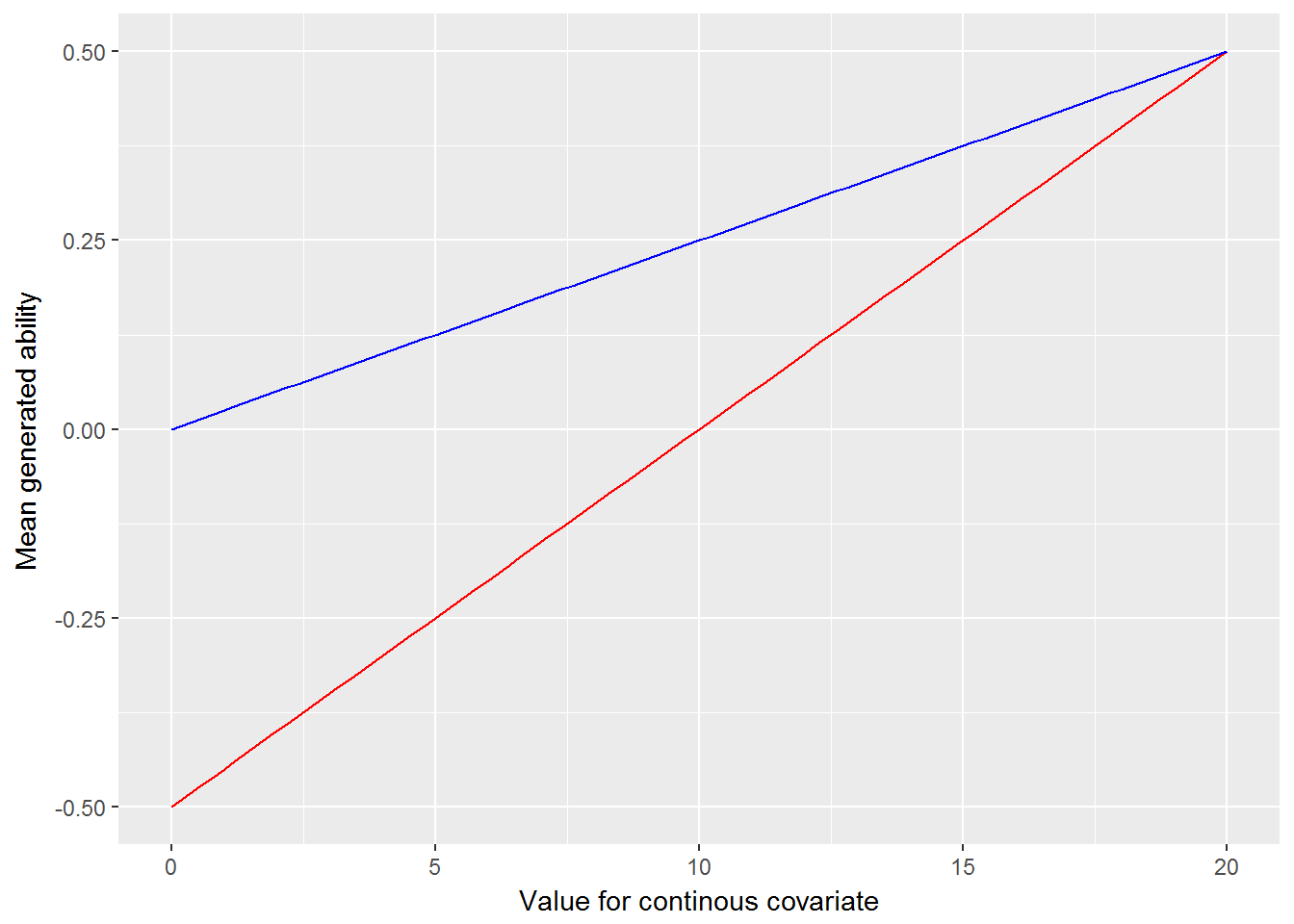Mean of generated abilities as a function of the continuous covariate. A line is shown separately for the two groups identified by the binary variable.

The simulated dataset is next fit with Stan using irt_stan() from the edstan package. irt_stan() is merely a wrapper for stan() in rstan. Using 1,000 posterior draws per chain may be somewhat excessive as we are mainly interested in the posterior means of the parameters. However, as parameter recovery will be evaulated using the 2.5th and 97.5th percentiles of the posterior, the large number of posterior samples is warranted.

# Fit model to simulated data using an edstan function
sim_rasch_fit <- irt_stan(sim_rasch_list, model = "rasch_latent_reg.stan",
chains = 4, iter = 1000)

The highest value for $$\hat R$$ was 1.004 for all parameters and the log posterior, suggesting that the chains have converged. The Stan model is evaluated in terms of its ability to recover the generating values of the parameters. The R code below prepares a plot in which the points indicate the difference between the posterior means and generating values for the parameters of main interest. This difference is referred to as discrepancy. The lines indicate the 95% poster intervals for the difference, defined as the 2.5th and 97.5th percentiles of the posterior draws. Ideally, (nearly) all the 95% intervals would include zero.

# Get estimated and generating values for wanted parameters
rasch_generating_values <- c(beta, lambda, sigma)
rasch_estimated_values <- summary(sim_rasch_fit,
pars = c("beta", "lambda", "sigma"),
probs = c(.025, .975))
rasch_estimated_values <- rasch_estimated_values[["summary"]]

# Make a data frame of the discrepancies
rasch_discrep <- data.frame(par = rownames(rasch_estimated_values),
mean = rasch_estimated_values[, "mean"],
p025 = rasch_estimated_values[, "2.5%"],
p975 = rasch_estimated_values[, "97.5%"],
gen = rasch_generating_values)
rasch_discrep$par <- with(rasch_discrep, factor(par, rev(par))) rasch_discrep$lower <- with(rasch_discrep, p025 - gen)
rasch_discrep$middle <- with(rasch_discrep, mean - gen) rasch_discrep$upper <- with(rasch_discrep, p975 - gen)

# Plot the discrepancies
ggplot(rasch_discrep) +
aes(x = par, y = middle, ymin = lower, ymax = upper) +
scale_x_discrete() +
labs(y = "Discrepancy", x = NULL) +
geom_abline(intercept = 0, slope = 0, color = "white") +
geom_linerange() +
geom_point(size = 2) +
theme(panel.grid = element_blank()) +
coord_flip()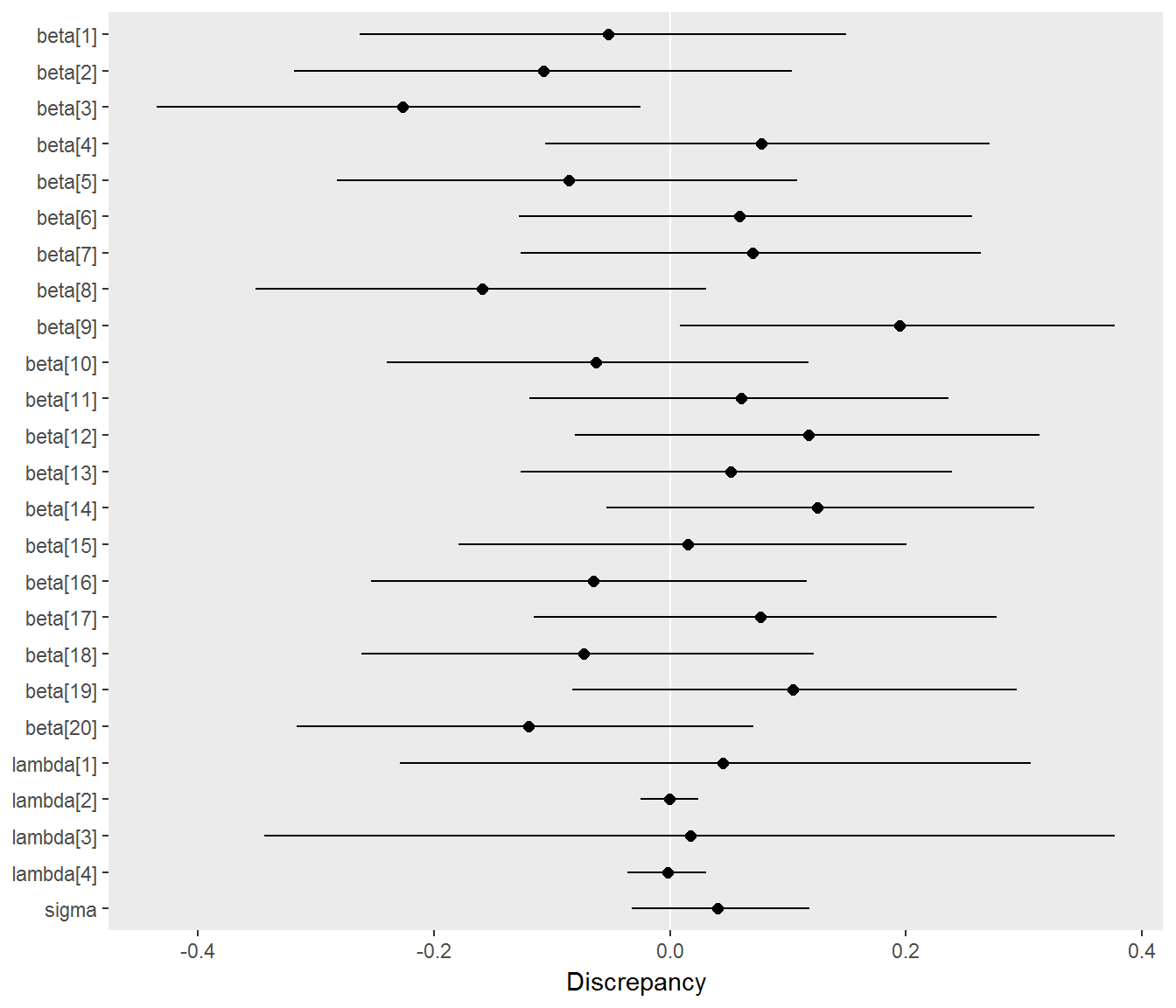Discrepancies between estimated and generating parameters. Points indicate the difference between the posterior means and generating values for a parameter, and horizontal lines indicate 95% posterior intervals for the difference. Most of the discrepancies are about zero, indicating that Stan successfully recovers the true parameters.

# 2 Two-parameter logistic model with latent regression

## 2.1 Overview of the model

The two-parameter logistic model (2PL) (Swaminathan and Gifford 1985) is an item response theory model that includes parameters for both the difficulty and discrimination of dichotomous items. The version presented includes a latent regression. However, the latent regression part of the model may be restricted to an intercept only, resulting in a regular 2PL.

$\mathrm{logit} [ \Pr(y_{ij} = 1 | \alpha_i, \beta_i, \theta_j) ] = \alpha_i \theta_j - \beta_i$ $\theta_j \sim \mathrm{N}(w_j' \lambda, 1)$

Many aspects of the 2PL are similar to the Rasch model described earlier. Parameters $$\beta_i$$, $$\theta_j$$, and $$\lambda$$ have the same interpretation, but the 2PL adds a discrimination parameter $$\alpha_i$$ and constrains the variance of $$\theta_j$$ to one. The prior $$\alpha_i \sim \mathrm{log~N}(1, 1)$$ is added, which is weakly informative but assumes positive discriminations. The same priors are placed on $$\beta_i$$ and $$\lambda$$, and the same constraint is placed on $$\beta_I$$.

## 2.2Stan code for the 2PL with latent regression

The Stan code for the 2PL is similar to that for the Rasch model except for the addition of the discrimination parameters.

# Print the latent regression 2PL model from the edstan package
twopl_latreg_file <- system.file("extdata/2pl_latent_reg.stan",
package = "edstan")
cat(readLines(twopl_latreg_file), sep = "\n")
functions {
matrix obtain_adjustments(matrix W) {
real min_w;
real max_w;
int minmax_count;
matrix[2, cols(W)] adj;
adj[1, 1] = 0;
adj[2, 1] = 1;
if(cols(W) > 1) {
for(k in 2:cols(W)) {                       // remaining columns
min_w = min(W[1:rows(W), k]);
max_w = max(W[1:rows(W), k]);
minmax_count = 0;
for(j in 1:rows(W))
minmax_count = minmax_count + W[j,k] == min_w || W[j,k] == max_w;
if(minmax_count == rows(W)) {       // if column takes only 2 values
adj[1, k] = mean(W[1:rows(W), k]);
adj[2, k] = (max_w - min_w);
} else {                            // if column takes > 2 values
adj[1, k] = mean(W[1:rows(W), k]);
adj[2, k] = sd(W[1:rows(W), k]) * 2;
}
}
}
return adj;
}
}
data {
int<lower=1> I;               // # questions
int<lower=1> J;               // # persons
int<lower=1> N;               // # observations
int<lower=1, upper=I> ii[N];  // question for n
int<lower=1, upper=J> jj[N];  // person for n
int<lower=0, upper=1> y[N];   // correctness for n
int<lower=1> K;               // # person covariates
matrix[J,K] W;                // person covariate matrix
}
transformed data {
matrix[2,K] adj;               // values for centering and scaling covariates
matrix[J,K] W_adj;             // centered and scaled covariates
adj = obtain_adjustments(W);
for(k in 1:K) for(j in 1:J)
W_adj[j,k] = (W[j,k] - adj[1,k]) / adj[2,k];
}
parameters {
vector<lower=0>[I] alpha;
vector[I-1] beta_free;
vector[J] theta;
vector[K] lambda_adj;
}
transformed parameters {
vector[I] beta;
beta[1:(I-1)] = beta_free;
beta[I] = -1*sum(beta_free);
}
model {
alpha ~ lognormal(1, 1);
target += normal_lpdf(beta | 0, 3);
lambda_adj ~ student_t(3, 0, 1);
theta ~ normal(W_adj*lambda_adj, 1);
y ~ bernoulli_logit(alpha[ii].*theta[jj] - beta[ii]);
}
generated quantities {
vector[K] lambda;
lambda[2:K] = lambda_adj[2:K] ./ to_vector(adj[2,2:K]);
lambda = W_adj[1, 1:K]*lambda_adj[1:K] - W[1, 2:K]*lambda[2:K];
}

## 2.3 Simulation for parameter recovery

The Stan model is fit to a simulated dataset to evaluate it’s ability to recover the generating parameter values. The R code that follows simulates a dataset conforming to the model. The item difficulties and some other elements are borrowed from the Rasch model simulation.

# Set alpha, and otherwise use parameters from the previous simulation
alpha <- rep(c(.8, 1, 1.2, 1.4),  length.out = I)

# Calculate or sample remaining variables and parameters where needed
twopl_theta <-  rnorm(J, W %*% matrix(lambda), 1)
twopl_eta <- alpha[ii]*twopl_theta[jj] - beta[ii]
twopl_y <- rbinom(N, size = 1, prob = boot::inv.logit(twopl_eta))

# Assemble the data list using an edstan function
sim_2pl_list <- irt_data(y = twopl_y, ii = ii, jj = jj,
covariates = as.data.frame(W),
formula = NULL)

The simulated dataset is next fit with Stan using irt_stan() from the edstan package.

# Fit model to simulated data using an edstan function
sim_2pl_fit <- irt_stan(sim_2pl_list, model = "2pl_latent_reg.stan",
chains = 4, iter = 1000)

The highest value for $$\hat R$$ was 1.01 for all parameters and the log posterior. The Stan model is evaluated in terms of its ability to recover the generating values of the parameters. The R code below prepares a plot in which the points indicate the difference between the posterior means and generating values for the parameters of main interest. This difference is referred to as discrepancy. The lines indicate the 95% poster intervals for the difference, defined as the 2.5th and 97.5th percentiles of the posterior draws. Ideally, (nearly) all the 95% intervals would include zero.

# Get estimated and generating values for wanted parameters
twopl_generating_values <- c(alpha, beta, lambda)
twopl_estimated_values <- summary(sim_2pl_fit,
pars = c("alpha", "beta", "lambda"),
probs = c(.025, .975))
twopl_estimated_values <- twopl_estimated_values[["summary"]]

# Make a data frame of the discrepancies
twopl_discrep <- data.frame(par = rownames(twopl_estimated_values),
mean = twopl_estimated_values[, "mean"],
p025 = twopl_estimated_values[, "2.5%"],
p975 = twopl_estimated_values[, "97.5%"],
gen = twopl_generating_values)
twopl_discrep$par <- with(twopl_discrep, factor(par, rev(par))) twopl_discrep$lower <- with(twopl_discrep, p025 - gen)
twopl_discrep$middle <- with(twopl_discrep, mean - gen) twopl_discrep$upper <- with(twopl_discrep, p975 - gen)

# Plot the discrepancies
ggplot(twopl_discrep) +
aes(x = par, y = middle, ymin = lower, ymax = upper) +
scale_x_discrete() +
labs(y = "Discrepancy", x = NULL) +
geom_abline(intercept = 0, slope = 0, color = "white") +
geom_linerange() +
geom_point(size = 2) +
theme(panel.grid = element_blank()) +
coord_flip()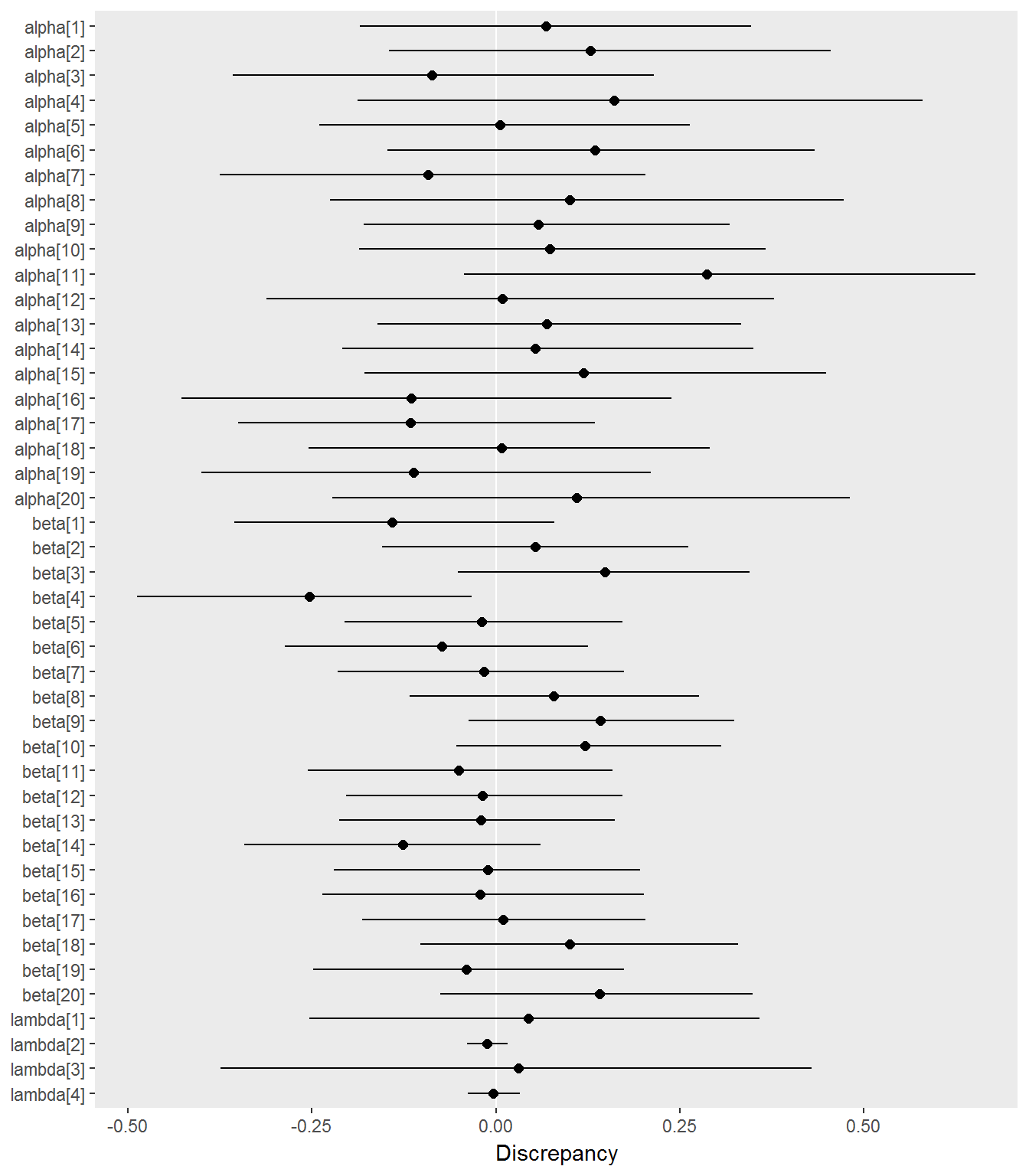Discrepancies between estimated and generating parameters. Points indicate the difference between the posterior means and generating values for a parameter, and horizontal lines indicate 95% posterior intervals for the difference. Most of the discrepancies are about zero, indicating that Stan successfully recovers the true parameters.

# 3 Example application

## 3.1 Data

The example data are from the The First International Mathematics Study (Husen and others 1967; Postlethwaite 1967). The data include information about student gender and country (Australia or Japan). For convenience, only a subset of the full data are used.

# Attach the example dataset. The TAM package is required.
data(data.fims.Aus.Jpn.scored, package = "TAM")

# Subset the full data
select <- floor(seq(from = 1, to = nrow(data.fims.Aus.Jpn.scored),
length.out = 500))
subsetted_df <- data.fims.Aus.Jpn.scored[select, ]
str(subsetted_df)
## 'data.frame':    500 obs. of  16 variables:
##  $SEX : int 1 1 2 2 1 1 2 2 2 1 ... ##$ M1PTI1 : num  1 1 1 0 0 1 1 1 1 1 ...
##  $M1PTI2 : num 0 0 0 0 0 1 1 1 1 1 ... ##$ M1PTI3 : num  1 1 1 0 1 1 0 1 1 0 ...
##  $M1PTI6 : num 1 0 0 1 0 0 0 1 1 0 ... ##$ M1PTI7 : num  0 0 0 0 0 1 0 0 0 0 ...
##  $M1PTI11: num 1 0 0 0 0 0 1 1 1 0 ... ##$ M1PTI12: num  0 0 0 0 0 1 0 0 1 1 ...
##  $M1PTI14: num 0 0 1 0 0 1 0 1 1 1 ... ##$ M1PTI17: num  1 0 0 0 0 1 0 1 1 0 ...
##  $M1PTI18: num 0 0 1 1 0 0 1 1 0 1 ... ##$ M1PTI19: num  0 0 0 0 0 1 0 0 0 0 ...
##  $M1PTI21: num 0 0 0 0 0 0 0 0 0 0 ... ##$ M1PTI22: num  0 0 0 0 0 1 0 0 0 0 ...
##  $M1PTI23: num 1 1 1 1 0 1 1 1 0 0 ... ##$ country: int  1 1 1 1 1 1 1 1 1 1 ...

The dataset is next divided into an item response matrix and a matrix of student covariates.

# Extract the response matrix
response_matrix <- as.matrix(subsetted_df[, grepl("^M1", names(subsetted_df))])
dim(response_matrix)
##  500  14
# Set up a data frame of person covariates
covariates <- data.frame(male = as.numeric(subsetted_df$SEX == 2), japan = as.numeric(subsetted_df$country == 2))
table(covariates)
##     japan
## male   0   1
##    0 181  80
##    1 158  81

500 students responded to 4 dichotomously scored items. The data contain no missing values. The two matrices are converted to a list suitable for the Stan model.

# Assemble the data list using an edstan function
ex_list <- irt_data(response_matrix = response_matrix,
covariates = covariates,
formula = ~ male*japan)

## 3.2 Rasch model results

The Rasch model is fit to the data list.

# Fit the Rasch model model using an edstan function
ex_rasch_fit <- irt_stan(ex_list, model = "rasch_latent_reg.stan",
chains = 4, iter = 300)

As discussed above, convergence of the chains is assessed for every parameter, and also the log posterior density, using $$\hat{R}$$.

# Plot of convergence statistics using an edstan function
stan_columns_plot(ex_rasch_fit)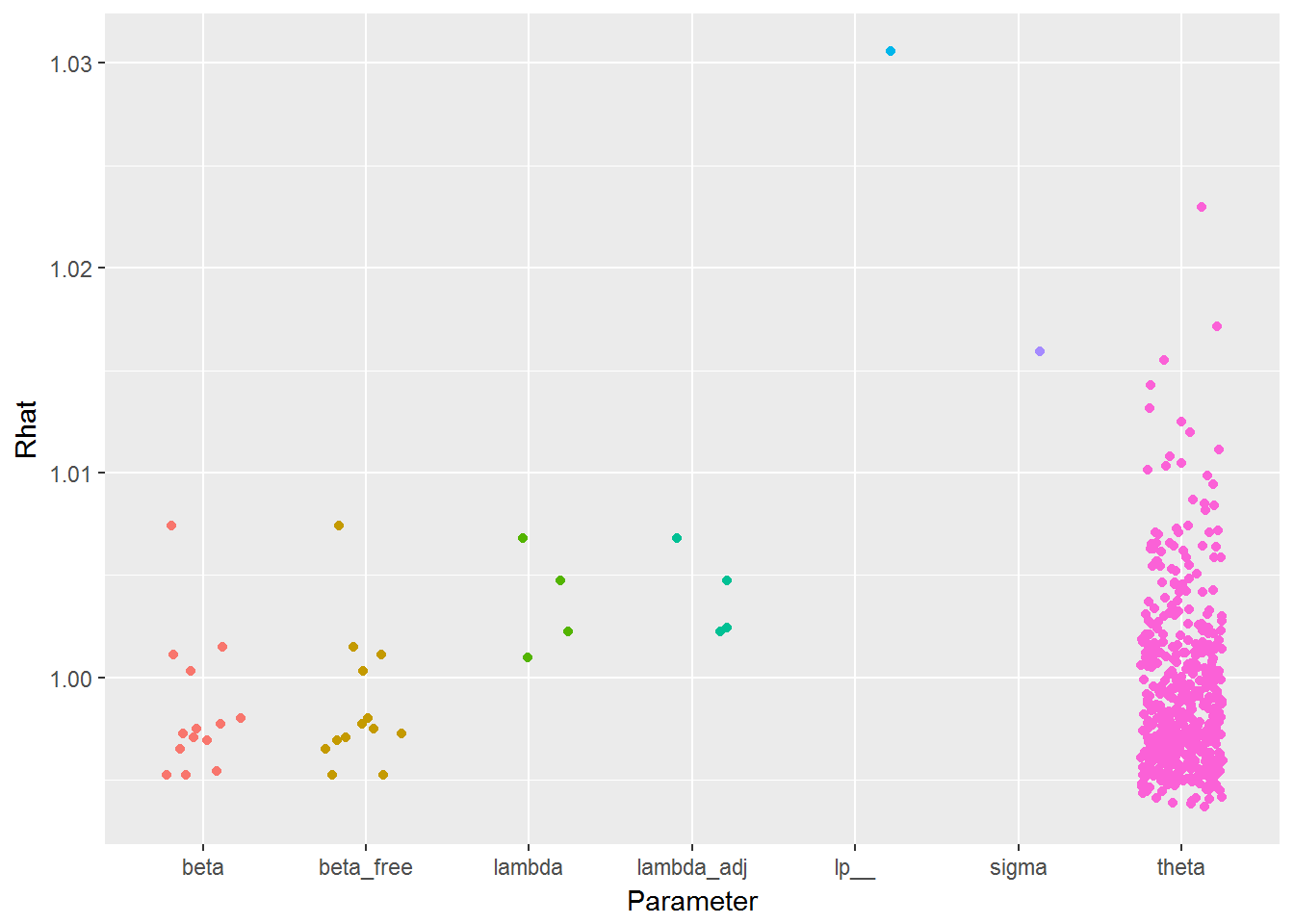Convergence statistics ($$\hat{R}$$) by parameter for the example. All values should be less than 1.1 to infer convergence. Horizontal jitter is applied to the points.

Next we view summaries of the parameter posteriors.

# View table of parameter posteriors using an edstan function
print_irt_stan(ex_rasch_fit)
## Inference for Stan model: rasch_latent_reg.
## 4 chains, each with iter=300; warmup=150; thin=1;
## post-warmup draws per chain=150, total post-warmup draws=600.
##
##            mean se_mean   sd  2.5%   25%   50%   75% 97.5% n_eff Rhat
## beta   -1.61    0.00 0.11 -1.84 -1.69 -1.61 -1.54 -1.40   600 1.00
## beta   -1.43    0.00 0.11 -1.64 -1.51 -1.43 -1.36 -1.22   600 1.00
## beta   -2.03    0.01 0.13 -2.27 -2.12 -2.02 -1.93 -1.77   600 1.00
## beta   -0.43    0.00 0.10 -0.62 -0.50 -0.43 -0.37 -0.25   445 1.01
## beta    1.84    0.00 0.12  1.61  1.77  1.84  1.92  2.07   600 1.00
## beta   -1.58    0.00 0.11 -1.78 -1.66 -1.59 -1.51 -1.38   600 1.00
## beta    0.82    0.00 0.10  0.61  0.75  0.82  0.89  1.02   509 1.00
## beta    0.35    0.00 0.09  0.18  0.29  0.35  0.42  0.53   577 1.00
## beta    1.07    0.00 0.10  0.87  1.00  1.06  1.13  1.27   600 1.00
## beta  -0.50    0.00 0.10 -0.68 -0.56 -0.49 -0.43 -0.31   600 1.00
## beta   1.60    0.00 0.11  1.39  1.53  1.60  1.68  1.84   600 1.00
## beta   1.29    0.00 0.10  1.10  1.21  1.28  1.36  1.49   481 1.00
## beta   1.76    0.00 0.12  1.54  1.68  1.75  1.83  1.99   600 1.00
## beta  -1.14    0.00 0.11 -1.34 -1.21 -1.15 -1.07 -0.93   600 1.00
## lambda -0.37    0.00 0.08 -0.53 -0.43 -0.37 -0.32 -0.22   412 1.00
## lambda  0.10    0.01 0.12 -0.13  0.01  0.09  0.18  0.33   380 1.01
## lambda  1.04    0.01 0.14  0.76  0.94  1.04  1.14  1.32   311 1.00
## lambda -0.25    0.01 0.21 -0.64 -0.38 -0.26 -0.10  0.19   317 1.00
## sigma      0.89    0.00 0.05  0.78  0.86  0.89  0.92  0.99   198 1.02
##
## Samples were drawn using NUTS(diag_e) at Mon Mar 06 14:25:42 2017.
## For each parameter, n_eff is a crude measure of effective sample size,
## and Rhat is the potential scale reduction factor on split chains (at
## convergence, Rhat=1).

A Rasch model without the latent regression could be fit by changing the person covariate matrix to include only an intercept term. Shown below is how this may be done for the example data.

# Fit the example data without latent regression
noreg_list <- ex_list
noreg_list$W <- matrix(1, nrow = ex_list$J, ncol = 1)
noreg_list\$K <- 1
noreg_fit <- stan(file = "rasch_latent_reg.stan",
data = noreg_list, chains = 4, iter = 300)

## 3.3 Two parameter logistic model results

The 2PL is fit to the data list.

# Fit the 2PL using an edstan function
ex_2pl_fit <- irt_stan(ex_list, model = "2pl_latent_reg.stan",
chains = 4, iter = 300)

As discussed above, convergence of the chains is assessed for every parameter, and also the log posterior density, using $$\hat{R}$$.

# Plot of convergence statistics using an edstan function
stan_columns_plot(ex_2pl_fit)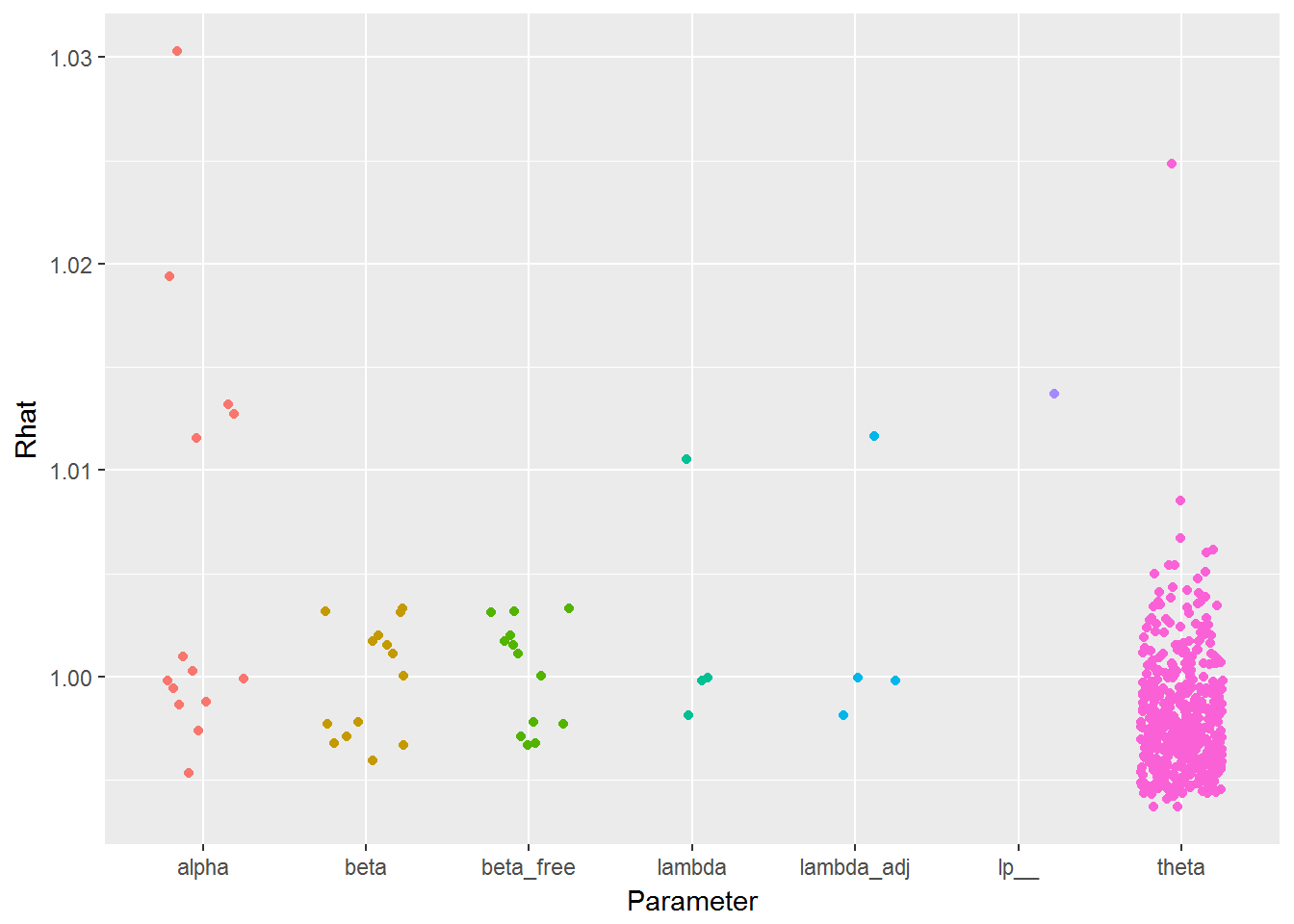Convergence statistics ($$\hat{R}$$) by parameter for the example. All values should be less than 1.1 to infer convergence. Horizontal jitter is applied to the points.

Next we view summaries of the parameter posteriors.

# View table of parameter posteriors using an edstan function
print_irt_stan(ex_2pl_fit, ex_list)
## Inference for Stan model: 2pl_latent_reg.
## 4 chains, each with iter=300; warmup=150; thin=1;
## post-warmup draws per chain=150, total post-warmup draws=600.
##
##              mean se_mean   sd  2.5%   25%   50%   75% 97.5% n_eff Rhat
## Item 1: M1PTI1
##   alpha   0.71    0.01 0.14  0.47  0.61  0.71  0.80  0.99   600 1.00
##   beta   -1.54    0.01 0.12 -1.82 -1.62 -1.54 -1.47 -1.31   600 1.00
## Item 2: M1PTI2
##   alpha   1.49    0.01 0.24  1.10  1.32  1.47  1.65  2.03   522 1.00
##   beta   -1.79    0.01 0.19 -2.22 -1.92 -1.78 -1.65 -1.48   600 1.00
## Item 3: M1PTI3
##   alpha   1.16    0.01 0.21  0.78  1.02  1.16  1.30  1.58   600 1.03
##   beta   -2.25    0.01 0.21 -2.69 -2.39 -2.25 -2.11 -1.86   600 1.00
## Item 4: M1PTI6
##   alpha   1.24    0.01 0.18  0.92  1.13  1.24  1.36  1.62   600 1.01
##   beta   -0.54    0.00 0.11 -0.74 -0.62 -0.55 -0.47 -0.32   600 1.00
## Item 5: M1PTI7
##   alpha   1.87    0.02 0.26  1.36  1.70  1.85  2.02  2.49   284 1.01
##   beta    2.42    0.01 0.22  1.99  2.28  2.42  2.56  2.90   600 1.00
## Item 6: M1PTI11
##   alpha   1.42    0.01 0.21  1.03  1.27  1.41  1.56  1.84   600 1.00
##   beta   -1.94    0.01 0.18 -2.32 -2.05 -1.93 -1.82 -1.59   600 1.00
## Item 7: M1PTI12
##   alpha   0.48    0.00 0.11  0.29  0.40  0.48  0.55  0.70   600 1.00
##   beta    0.73    0.00 0.10  0.54  0.67  0.73  0.80  0.93   600 1.00
## Item 8: M1PTI14
##   alpha   0.31    0.00 0.09  0.15  0.25  0.31  0.37  0.49   600 1.00
##   beta    0.31    0.00 0.09  0.13  0.25  0.31  0.37  0.49   600 1.00
## Item 9: M1PTI17
##   alpha   1.22    0.01 0.17  0.91  1.10  1.21  1.32  1.61   600 1.00
##   beta    1.12    0.00 0.12  0.93  1.03  1.12  1.20  1.35   600 1.00
## Item 10: M1PTI18
##   alpha  0.67    0.01 0.12  0.44  0.59  0.66  0.74  0.91   531 1.00
##   beta  -0.47    0.00 0.10 -0.66 -0.53 -0.47 -0.40 -0.28   600 1.00
## Item 11: M1PTI19
##   alpha  2.23    0.03 0.37  1.65  1.97  2.20  2.43  3.06   132 1.02
##   beta   2.31    0.02 0.25  1.88  2.13  2.30  2.46  2.87   268 1.00
## Item 12: M1PTI21
##   alpha  0.18    0.00 0.07  0.06  0.12  0.17  0.22  0.32   600 1.01
##   beta   1.12    0.00 0.12  0.90  1.03  1.11  1.19  1.38   600 1.00
## Item 13: M1PTI22
##   alpha  1.29    0.01 0.19  0.96  1.17  1.28  1.42  1.67   600 1.00
##   beta   1.94    0.01 0.17  1.63  1.83  1.93  2.04  2.29   600 1.00
## Item 14: M1PTI23
##   alpha  1.42    0.01 0.22  1.01  1.27  1.41  1.55  1.89   600 1.00
##   beta  -1.42    0.01 0.15 -1.69 -1.52 -1.41 -1.32 -1.13   600 1.00
## Ability distribution
##   lambda -0.43    0.00 0.09 -0.61 -0.49 -0.42 -0.36 -0.25   416 1.01
##   lambda  0.07    0.01 0.13 -0.18 -0.02  0.07  0.15  0.31   600 1.00
##   lambda  1.17    0.01 0.16  0.86  1.06  1.15  1.27  1.50   600 1.00
##   lambda -0.28    0.01 0.21 -0.70 -0.41 -0.27 -0.16  0.14   600 1.00
##
## Samples were drawn using NUTS(diag_e) at Mon Mar 06 14:25:56 2017.
## For each parameter, n_eff is a crude measure of effective sample size,
## and Rhat is the potential scale reduction factor on split chains (at
## convergence, Rhat=1).